# Quantitative Aptitude Questions (Data Interpretation) for SBI Clerk Mains 2018 Day-164

Dear Readers, SBI is conducting Online Examination for the recruitment of clerical cadre To enrich your preparation here we have providing new series of Data Interpretation – Quantitative Aptitude Questions. Candidates those who are appearing in SBI Clerk Mains Exams can practice these Quantitative Aptitude average questions daily and make your preparation effective.

[WpProQuiz 3030]

Click “Start Quiz” to attend these Questions and view Solutions

Directions (1-5): The two-line graph shows the distance travelled by the five different boats with the stream and against the stream in same time and the bar chart shows speed of stream.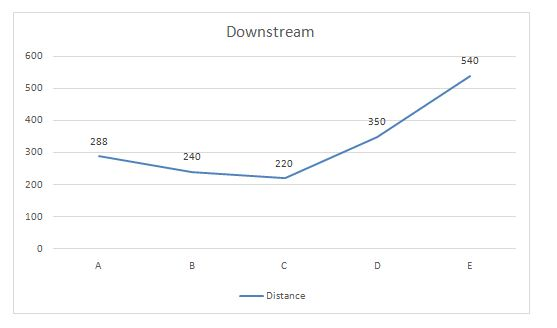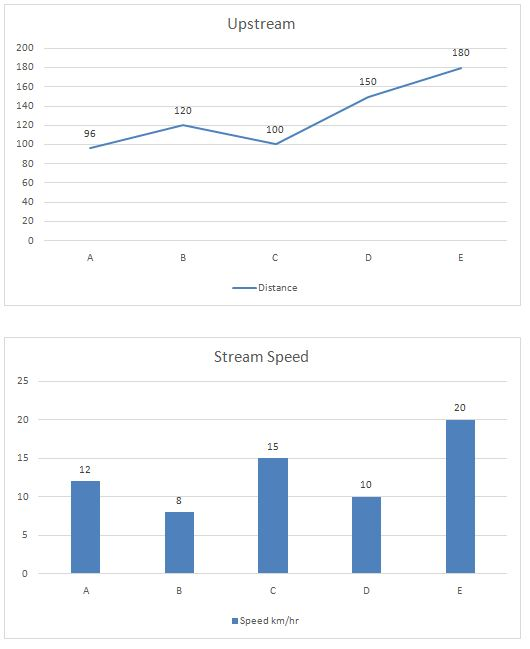1) Find out the speed of Boat B & D together in still water is approximately how much percentage more/less than that of speed of stream of the same boats together?

a) 70 %

b) 170%

c) 60%

d) 68%

e) 150%

2) It is known that ratio of the speed of the Boat B to Boat F in the still water is 4:5. If it’s given that Boat F travels 126 km distance with the stream, and 81 km against the stream in 7 hr 30 min, what is the speed of stream for Boat F?

a) 14

b) 13

c) 15

d) 12

e) 18

3) It’s known that distance between the two-point M & N is 210 km. Boat E travels from point M to N and Comes back. What is the time taken by Boat E in travelling total distance?

a) 12

b) 14

c) 18

d) 17

e) 16

4) Captain of the Boat thought of increasing the speed of Boat C and so in still water speed of boat is increased by 10% and stream also started flowing fast, increasing its speed by 20% due to wind. Find the time taken by the Boat C to cover 91km distance against the stream.

a) 7

b) 8.5

c) 3.5

d) 6.2

e) None

5) Find the ratio of the speed of Boats A and B together in still water to the speed of Boats D and E together in still water.

a) 48:55

b) 48:65

c) 43:33

d) 46:34

e) 44:34

Directions (6-10): Study the following information carefully and answer the questions given below: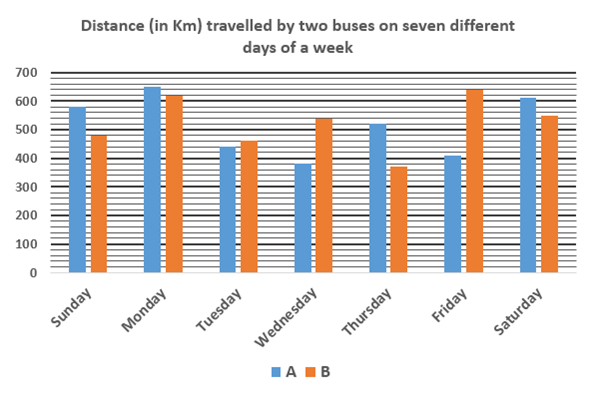The following table represents the ratios of distances travelled by bus A and bus C on seven different days.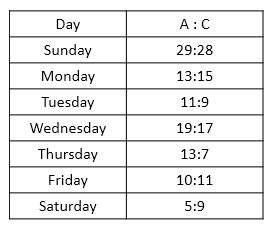6) Distance travelled by C on Tuesday is what percent of the distance travelled by C on Saturday?

a) 27.12%

b) 37.12%

c) 32.78%

d) 29.45%

e) None of these

7) Find the respective ratio of the distance travelled by A on Monday and Wednesday together and distance travelled by B on the same days.

a) 117:104

b) 103:116

c) 104:117

d) 116:103

e) None of these

8) Find the difference between distance travelled by A in all the days together and distance travelled by B in all the days together.

a) 60 km

b) 50 km

c) 40 km

d) 70 km

e) None of these

9) Distance travelled by C on Friday is what percent more/less than the distance travelled by B on Friday.

a) 29.53% more

b) 12.23% less

c) 12.23% more

d) 29.53% less

e) None of these

10) Find the sum of the distance travelled by C throughout the week.

a) 3567 Km

b) 4533 Km

c) 5432 Km

d) 3839 Km

e) None of these

Directions (1-5):

Boat A:

Let us take speed of boat A in still water be x kmph

Given,

288/(x+12) = 96/(x-12)

3/(x+12) = 1/(x-12)

3x-36 = x+12

2x = 48 => x= 24 kmph

Speed of boat A in still water is 24 kmph

Boat B:

Let us take speed of boat B in still water be x kmph

Given,

240/(x+8) = 120/(x-8)

2/(x+8) = 1/(x-8)

X+8 = 2x-16=> x= 24 kmph

Speed of boat B in still water is 24 kmph

Boat C:

Let us take speed of boat C in still water be x kmph

Given,

220/(x+15) = 100/(x-15)

11/(x+15) = 5/(x-15)

11x-165 = 5x+75

6x = 240 => x= 40 kmph

Speed of boat C in still water is 40 kmph

Boat D:

Let us take speed of boat D in still water be x kmph

Given,

350/(x+10) = 150/(x-10)

7/(x+10) = 3/(x-10)

7x-70 = 3x+30

4x = 100 => x= 25 kmph

Speed of boat C in still water is 25 kmph

Boat E:

Let us take speed of boat E in still water be x kmph

Given,

540/(x+20) = 180/(x-20)

3/(x+20) = 1/(x-20)

3x-60 = x+20

2x = 80 => x= 40 kmph

Speed of Boat B and D, in still water together = (24 + 25) = 49 km/h

Speed of stream of Boat B and D together = 18 km/h

% more =    (49 – 18)/18 × 100

=       31/18 × 100 = 172.22% ≈ 170%

2)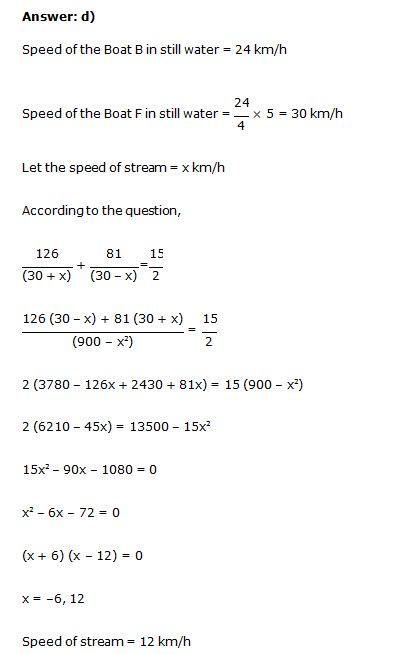3)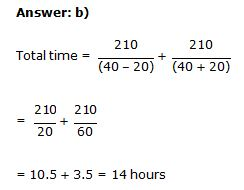Speed of Boat C in still water = 40 × 110% = 44 km/h

Speed of stream = 15 × 120% = 18 km/h

Time taken by Boat C to cover the distance of 91 km upstream = 91/ (44 – 18)

= 91/ 26 = 3.5 hours

Ratio = (24 + 24) : (25 + 40)

= 48 : 65

Direction (6-10)

Distance travelled by C on Tuesday = 9/11 x 440 = 360 Km

Distance travelled by C on Saturday = 9/5 x 610 = 1098 Km

Required percentage = 360/1098 x 100 = 32.78%

Distance travelled by A on Monday and Wednesday together = 650 + 380 = 1030 Km

Distance travelled by B on Monday and Wednesday together = 620 + 540 = 1160 km

Required ratio = 1030:1160

= 103:116

Distance travelled by A in all the days together = 580 + 650 + 440 + 380 + 520 + 410 + 610 = 3590 km

Distance travelled by B in all the days together = 480 + 620 + 460 + 540 + 370 + 640 + 550 = 3660 km

Required difference = 3660 – 3590 = 70 km

Distance travelled by C on Friday = 11/10 x 410 = 451 km

Required percentage = (640 – 451)/640 x 100

= 189/640 x 100

= 29.53% less

Distance travelled by C on Sunday = 28/29 x 580 = 560 Km

Distance travelled by C on Monday = 15/13 x 650 = 750 Km

Distance travelled by C on Tuesday = 9/11 x 440 = 360 Km

Distance travelled by C on Wednesday= 17/19 x 380 = 340 Km

Distance travelled by C on Thursday= 7/13 x 520 = 280 Km

Distance travelled by C on Friday = 11/10 x 410 = 451 Km

Distance travelled by C on Saturday = 9/5 x 610 = 1098 Km

Required sum = 560 + 750 + 360 + 340 + 280 + 451 + 1098 = 3839 Km

Daily Practice Test Schedule | Good Luck

 Topic Daily Publishing Time Daily News Papers & Editorials 8.00 AM Current Affairs Quiz 9.00 AM Quantitative Aptitude “20-20” 11.00 AM Vocabulary (Based on The Hindu) 12.00 PM General Awareness “20-20” 1.00 PM English Language “20-20” 2.00 PM Reasoning Puzzles & Seating 4.00 PM Daily Current Affairs Updates 5.00 PM Data Interpretation / Application Sums (Topic Wise) 6.00 PM Reasoning Ability “20-20” 7.00 PM English Language (New Pattern Questions) 8.00 PM